# HSSlive: Plus One & Plus Two Notes & Solutions for Kerala State Board

## AP Board Class 7 Maths Chapter 8 Congruence of Triangles Ex 3 Textbook Solutions PDF: Download Andhra Pradesh Board STD 7th Maths Chapter 8 Congruence of Triangles Ex 3 Book AnswersAP Board Class 7 Maths Chapter 8 Congruence of Triangles Ex 3 Textbook Solutions PDF: Download Andhra Pradesh Board STD 7th Maths Chapter 8 Congruence of Triangles Ex 3 Book Answers

## Andhra Pradesh State Board Class 7th Maths Chapter 8 Congruence of Triangles Ex 3 Books Solutions

 Board AP Board Materials Textbook Solutions/Guide Format DOC/PDF Class 7th Subject Maths Chapters Maths Chapter 8 Congruence of Triangles Ex 3 Provider Hsslive

2. Click on the Andhra Pradesh Board Class 7th Maths Chapter 8 Congruence of Triangles Ex 3 Answers.
3. Look for your Andhra Pradesh Board STD 7th Maths Chapter 8 Congruence of Triangles Ex 3 Textbooks PDF.
4. Now download or read the Andhra Pradesh Board Class 7th Maths Chapter 8 Congruence of Triangles Ex 3 Textbook Solutions for PDF Free.

## AP Board Class 7th Maths Chapter 8 Congruence of Triangles Ex 3 Textbooks Solutions with Answer PDF Download

Find below the list of all AP Board Class 7th Maths Chapter 8 Congruence of Triangles Ex 3 Textbook Solutions for PDF’s for you to download and prepare for the upcoming exams:

Question 1.
In following pairs of triangles, fmd the pairs which are congruent? Also, write the criterion of congruence.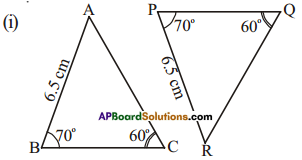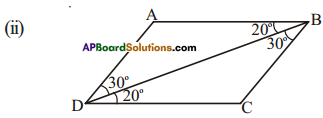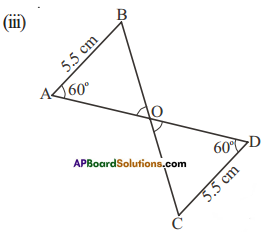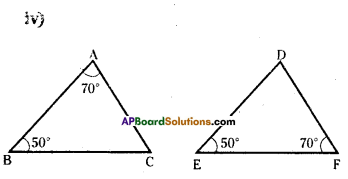Solution:AB = PR
∠B = ∠P
∠C = ∠Q
Now by angle sum property
∠A = ∠R
∴ By A.S.A, ΔABC ≅ ΔRPQ∠ABD = ∠CDB
DB = DBAB = CD
∠A = ∠D
∠B = ∠C (by angle sum properly
By A.S.A
ΔABO ≅ ΔDCOWe can say whether the triangles are congruent or not, at least one pair of sides should be given equal.

Question 2.
(i) Are ΔABC and ΔDCB congruent?
(ii) Are ΔAOB congruent to ΔDOC’?
Also identify the relation between corresponding elements and give reason for your answer.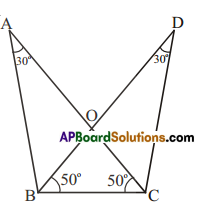Solution:
i) yes.
∠ACB = ∠DBC
BC = BC
∠ABC = ∠DCB (by angle sum property)
∴ ΔABC ≅ ΔDCB (A.S.A)

ii) yes
∠A = ∠D
∠ABO = ∠DCO (angle sum property)
AB = DC [From (i)]
∴ ΔAOB ≅ ΔDOC (A.S.A)
Otherwise ΔAOB and ΔDOC are similar by A.A.A. In congruent triangles corresponding parts
are equal.

## Andhra Pradesh Board Class 7th Maths Chapter 8 Congruence of Triangles Ex 3 Textbooks for Exam Preparations

Andhra Pradesh Board Class 7th Maths Chapter 8 Congruence of Triangles Ex 3 Textbook Solutions can be of great help in your Andhra Pradesh Board Class 7th Maths Chapter 8 Congruence of Triangles Ex 3 exam preparation. The AP Board STD 7th Maths Chapter 8 Congruence of Triangles Ex 3 Textbooks study material, used with the English medium textbooks, can help you complete the entire Class 7th Maths Chapter 8 Congruence of Triangles Ex 3 Books State Board syllabus with maximum efficiency.

## FAQs Regarding Andhra Pradesh Board Class 7th Maths Chapter 8 Congruence of Triangles Ex 3 Textbook Solutions

#### Can we get a Andhra Pradesh State Board Book PDF for all Classes?

Yes you can get Andhra Pradesh Board Text Book PDF for all classes using the links provided in the above article.

## Important Terms

Andhra Pradesh Board Class 7th Maths Chapter 8 Congruence of Triangles Ex 3, AP Board Class 7th Maths Chapter 8 Congruence of Triangles Ex 3 Textbooks, Andhra Pradesh State Board Class 7th Maths Chapter 8 Congruence of Triangles Ex 3, Andhra Pradesh State Board Class 7th Maths Chapter 8 Congruence of Triangles Ex 3 Textbook solutions, AP Board Class 7th Maths Chapter 8 Congruence of Triangles Ex 3 Textbooks Solutions, Andhra Pradesh Board STD 7th Maths Chapter 8 Congruence of Triangles Ex 3, AP Board STD 7th Maths Chapter 8 Congruence of Triangles Ex 3 Textbooks, Andhra Pradesh State Board STD 7th Maths Chapter 8 Congruence of Triangles Ex 3, Andhra Pradesh State Board STD 7th Maths Chapter 8 Congruence of Triangles Ex 3 Textbook solutions, AP Board STD 7th Maths Chapter 8 Congruence of Triangles Ex 3 Textbooks Solutions,
Share: